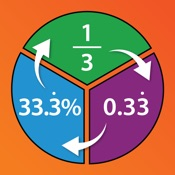# Converting Fractions, Decimals and Percentages - Lumos Educational App Store1
Price -\$2.57
\$2.57

#### DESCRIPTION:

Brought to you by Award-Winning educational publisher, Daydream Education, this fun and interactive app is packed full of engaging educational content and stimulating assessment activities that are appropriate for a wide range of ages and abilities. The Converting Fractions, Decimals and Percentages app breaks down difficult topics into manageable chunks of information to help improve users understanding of converting between fractions, decimals and percentages. Within the app users can learn and practice how to convert between fractions decimals and percentages. Each process is broken down

#### OVERVIEW:

Converting Fractions, Decimals and Percentages is a free educational mobile app By Chris Malcolm Ltd.It helps students in grades 3,4,5 practice the following standards 3.NF.2,4.NF.6,5.NF.A.1.

This page not only allows students and teachers download Converting Fractions, Decimals and Percentages but also find engaging Sample Questions, Videos, Pins, Worksheets, Books related to the following topics.

1. 3.NF.2 : Understand a fraction as a number on the number line; represent fractions on a number line diagram. (Grade 3 expectations in this domain are limited to fractions with denominators 2, 3, 4, 6, and 8.).

2. 4.NF.6 : Use decimal notation for fractions with denominators 10 or 100. For example, rewrite 0.62 as 62/100 ; describe a length as 0.62 meters; locate 0.62 on a number line diagram. (Grade 4 expectations in this domain are limited to fractions with denominators 2, 3, 4, 5, 6, 8, 10, 12, and 100.).

3. 5.NF.A.1 : Add and subtract fractions with unlike denominators (including mixed numbers) by replacing given fractions with equivalent fractions in such a way as to produce an equivalent sum or difference of fractions with like denominators. For example, 2/3 + 5/4 = 8/12 + 15/12 = 23/12. (In general, a/b + c/d = (ad + bc)/bd.) .

3
4
5

#### STANDARDS:

3.NF.2
4.NF.6
5.NF.A.1

Developer: Chris Malcolm Ltd

Software Version: 2

Category: Education

### RELATED APPSEdSearch WebSearch